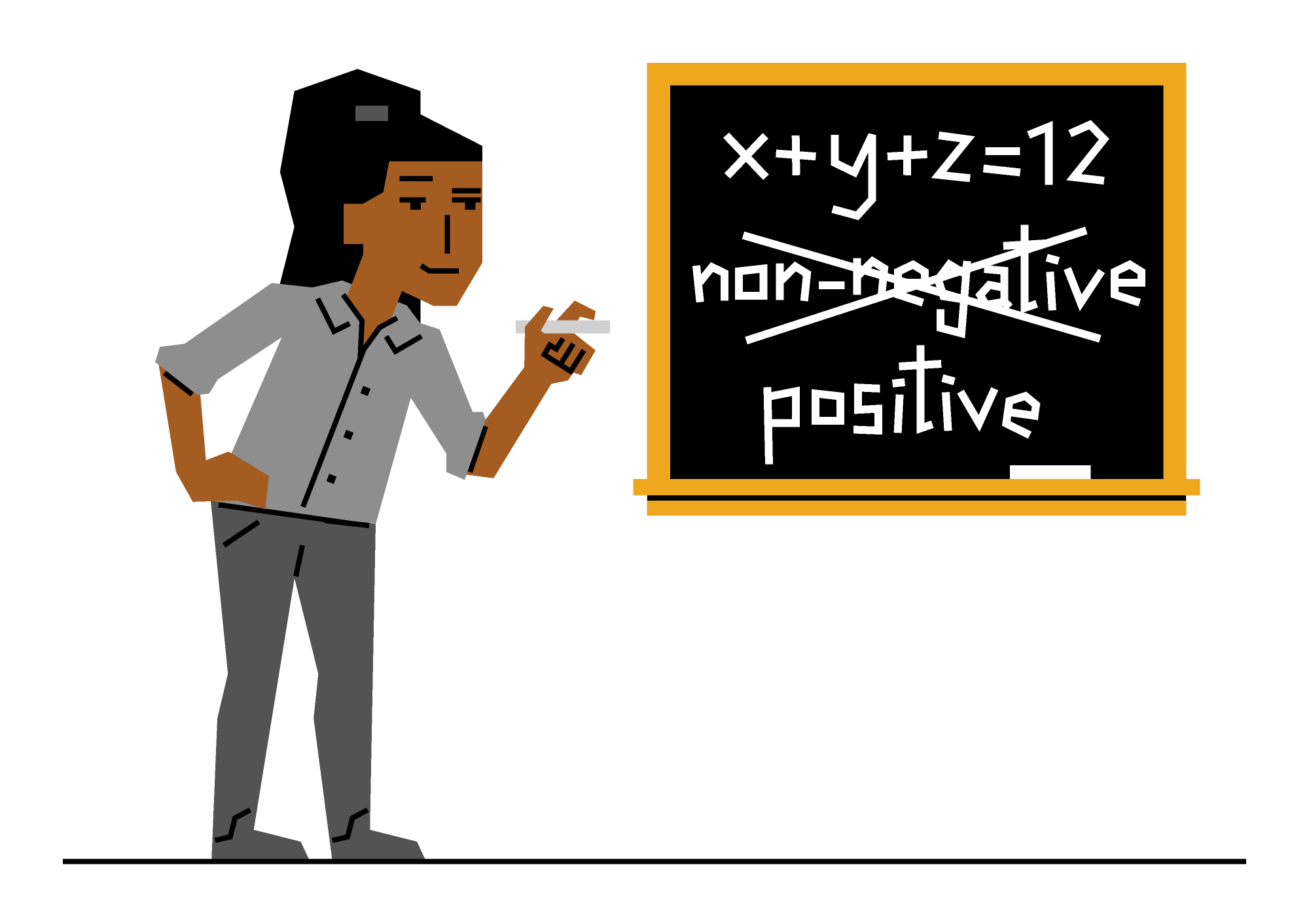Back

## A Test Question

Today, Pearl’s $9$ grandchildren are coming to visit! She loves to spoil them, so she opens her purse and finds $13$ dollar bills.

In how many different ways can Pearl distribute those dollars amongst her grandchildren? Keep reading to find out, or skip to today’s challenge for a similar problem.

As we’ll see, there are a lot of ways for Pearl to distribute her dollars! So, let’s start with a smaller example. Last week, Pearl’s $3$ favorite grandchildren visited, and at that time, she had $4$ dollar bills to give them. To visualize how they could be distributed, she laid them out in a row, along with some pencils to divide them into $3$ groups.

We’ll represent the dollars with stars $\large \star$ and divisions between groups with bars $\large{|}.$ One arrangement that Pearl found was $\large \star \; | \, \star \star \; | \; \star$ which represents $1$ dollar for the first grandchild, $2$ dollars for the second, and $1$ dollar for the third. Another arrangement was $\large \star \; | \: | \, \star \star \, \star$ which represents $1$ dollar for the first grandchild, $0$ dollars for the second, and $3$ dollars for the third.

To create $3$ groups, we need $2$ bars to separate the stars. So, to count the total number of arrangements into groups, we can count where in the line of stars and bars we can place those bars to define the groups.

With $4$ stars and $2$ bars, there are $6$ positions in the line. Generally, if we want to choose $k$ things from a set of $n$ things, there are $\binom n k = \frac{n!}{k!(n-k)!}$ ways to do so. So, by choosing which positions the $2$ bars take, we see there are $\binom 6 2 = \frac{6!}{2!(6-2)!} = 15$ ways to create $3$ groups of stars. That means Pearl had $15$ different ways to distribute $4$ dollar bills to her $3$ favorite grandchildren.

Now we can use this same method to count the number of ways for Pearl to distribute $13$ dollar bills amongst all $9$ of her grandchildren — we represent it with $13$ stars in a row, and we can divide them into $9$ groups using $8$ bars. Then there are $13+8=21$ positions available for the bars, and the rest must be filled with stars. That makes $\binom{21}{8} = 203940$ arrangements, or possible distributions of dollars!

Woah… hopefully Pearl has a way to narrow down the choices! Now, can you use stars and bars to solve today’s challenge?

# Today's Challenge

Leticia is writing a test question for her math students. She wants them to find solutions to the equation $x + y + z = 12,$ where $x, y,$ and $z$ must all be non-negative integers.If she changes the "non-negative" condition to "positive," then the number of solutions will decrease. By how many? $\\[-0.3em]$We are sunsetting our community features by July 2, 2021. Learn more here.
×

Problem Loading...

Note Loading...

Set Loading...